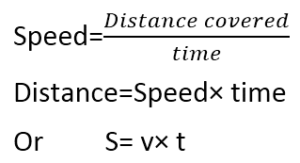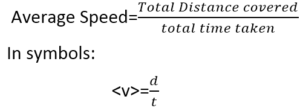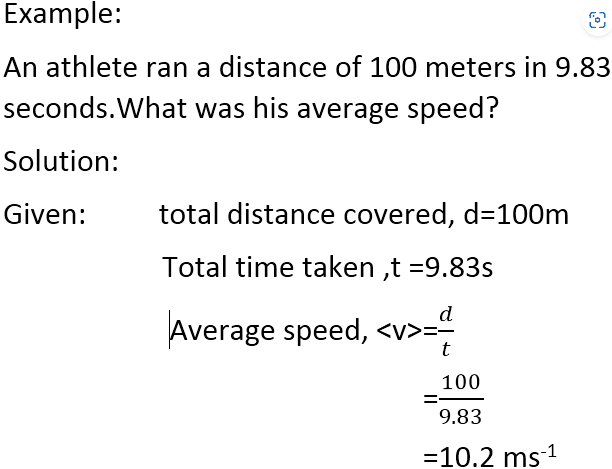Mechanics

# Definition of Speed In Physics with Examples

## What is speed in physics?

The definition of Speed in Physics is the distance covered by an object in units of time. In other words, the rate at which the object is moving is called speed. Unit time may be a second, an hour, a day, or a year. It tells us how fast or slow an object is moving. Speed is the scalar Quantity. SI unit of speed is m/s. Examples of Speed are given below in the post.

### How to Calculate Speed In Physics?

We can calculate Speed by using the Formula of Speed in Physics:Here S is the distance covered by the object, V is its speed, and t is the time taken by it. Distance is a scalar; therefore ‘speed is also a scalar quantity.SI unit of speed is meter per second, other units like kilometer per hour, miles per hour, feet per second are also used.

### Average Speed Definition:

Most objects or bodies do not move at a constant speed.For example, the MRT train starts from rest at a station, moves faster until it reaches a constant (or cruising) speed, and then slows down to a stop at the next station. It is, therefore, more useful to define average speed <v> rather than the actual speed v.

#### What is Formula for Average Speed?

Following Formula is used for Calculating Average Speed by dividing total distance covered by total time.

Thus:#### Average Speed Problems:## Examples of Speed

 Moving object Speed in ms-1 Speed in kmh-1 Earth rotating around the sun 30 000 108 000 Boeing 747B 300 1080 Car 16.7 60 Sprinter 10 36 MRT 12.5 45 Walking 2.5 9

The speed of sound in air at room temperature is about 330 m ⁄s or about 1200 km/h but the fastest speed is that of light in a vacuum is 3 × 10¤8 m/s.

### Difference between Uniform and non Uniform Speed

#### Uniform Speed:

The body has uniform speed if it covers equal distances in equal intervals of time however short the interval may be.

In the equation, S=v t  v is the average speed of a body during time t. It is because the speed of the body may be changing during the time interval t. However, if the speed of a body does not vary and has the same value then the body is said to possess uniform speed.

#### Non Uniform Speed:

“A body does not have uniform speed if it does not cover the equal distance with an equal interval of time.”
Related Topics:

### Related Articles

1. I merely wish to reveal to you that I am new to writing a blog and utterly loved your review. Probably I am likely to store your blog post . You definitely have fantastic article content. Be Grateful For it for giving out with us your favorite blog page

2. Amir Rauf says:

Great Website!